## Pages

Showing posts with label limits. Show all posts
Showing posts with label limits. Show all posts

# DIFFERENTIATION OF STANDARD FUNCTIONS

CBSE Class 11 - Mathematics - Limits and Derivatives Part-10

You have learnt theorems of differentiation part-9. Let us proceed further. Here is a list of differentiation of some standard functions: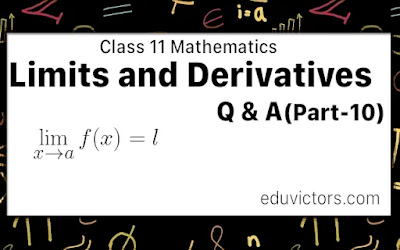# THEOREMS ON DIFFERENTIATION

CBSE Class 11 - Mathematics - Limits and Derivatives Part-9

You have learnt about finding derivatives using the first principle in part-7 and part-8. Let us proceed further. Here is a summary of theorems on differentiation.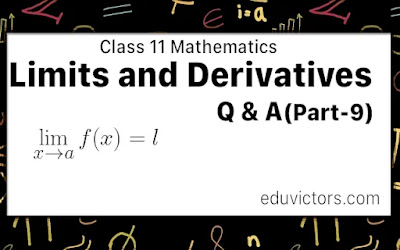# CBSE Class 11 - Mathematics - Limits and Derivatives Part-8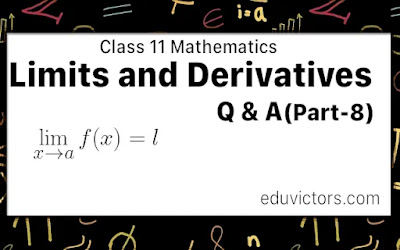Differentiation

In the previous post[Part-7], we learned about some important derivatives using the First Principle.

Let us talk about what differential co-efficient is?

Differential coefficient: Let y = f (x) be a continuous function of a variable quantity x, where x is independent and y. is a dependent variable quantity.# CBSE Class 11 - Mathematics - Limits and Derivatives Part-7

Derivatives Using First Principle

In the previous post[Part-6], we learned about the Derivative of a function at a point. Let us focus on some important derivatives using the First Principle.

Theorem 1: From the first principle, we have# Class 11 - Mathematics - Limits and Derivatives (Part-6) - Derivative of a Function At a Point

In the previous blog post Limits and derivatives Part-5, we learned about the limits. Let us understand the derivative of a function at a point.

Q1: What is the derivative of a function at a point?

Answer: Let y = f(x) is a continuous function. It means the value of y changes as the value of x changes.

At x = a, is a point in its domain of definittion. The derivative of f at a is defines as: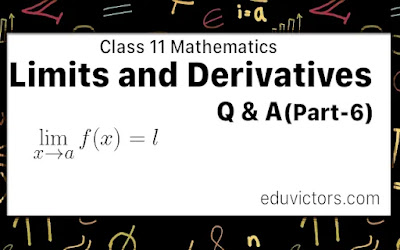# CBSE Class 11 - Mathematics - Limits and Derivatives Part-5

In the previous blog post Limits and derivatives Part-4, we learned about the  trigonometric limits. Let us solve other problems related to Limits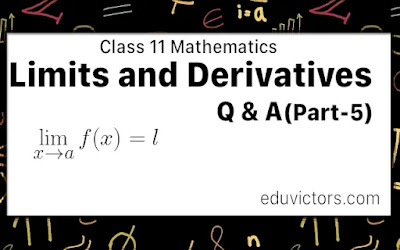# CBSE Class 11 - Mathematics - Limits and Derivatives Part-4- Limits of Trigonometric Functions

In the previous blog post Limits and derivatives Part-3, we learn about the algebra of limits. Now, let us study few theorems before we discuss about trignometric limits.

Theorem 1: Let f(x) and g(x) be two real valued functions with same domain such that f(x) ≤ g(x) for all x.
For some a, if $\lim_{x\rightarrow a} f(x)$ and $\lim_{x\rightarrow a} g(x)$ exist then $\lim_{x\rightarrow a} f(x) \leq \lim_{x\rightarrow a} g(x)$

Theorem 2: Sandwich Theorem or Squeeze Theorem
Let f(x) and g(x) be two real valued functions with same domain such that f(x) ≤ g(x) ≤ h(x) for all x in common domain.
For some a, if $\lim_{x\rightarrow a} f(x) = l = \lim_{x\rightarrow a} h(x)$, then $\lim_{x\rightarrow a} g(x) = l$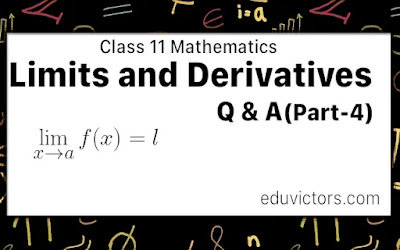# CBSE Class 11 - Mathematics - Limits and Derivatives

Part-3  Algebra of Limits

In the previous post Limits and derivatives Part-2, we get basic ideas of the algebra of limits and also learned about rules and properties of limits.

Let us try to solve few problems:

Q1: Evaluate the given limit $\lim_{r\rightarrow 1} \pi r^2$

Answer: $\lim_{r\rightarrow 1} \pi r^2 = \pi(1)^2 = \pi$# CBSE Class 11 - Mathematics - Limits and Derivatives (Part-2)

In the previous blog post Limits and derivatives Part-1 , we learn

$\lim_{x\rightarrow a} f(x) = l$ and it is called limit of the function f(x)

The two ways x could approach a number an either from left or from right, i.e., all the values of x near a could be less than a or could be greater than a.

In this case the right and left hand limits are different, and hence we say that the limit of f(x) as x tends to zero does not exist (even though the function is defined at 0).# CBSE Class 11 - Mathematics - Limits and Derivatives

Q1: Define Calculus.

Answer: Calculus is that branch of mathematics that mainly deals with the study of change in the value of a function as the points in the domain change.

👉Note: The chapter "Limits and Derivatives" is an introduction to Calculus.

👉Calculus is a Latin word meaning ‘pebble’. Ancient Romans used stones for counting.

Q2: Who are called pioneers of Calculus (who invented Calculus)?

Answer: Issac Newton (1642 - 1727) and G. W. Leibnitz(1646 - 1717).

Both of them were invented independently around the 17th century.

Q3: What is the meaning of 'x tends to a' or x → a?

Answer: When x tends to a (x  → a), x is nearly close to a but never equals to a.

e.g. x → 3 means the value of x maybe 2.99 or 2.999 or 2.999...9 is very close to 3 but not exactly equal to 3. Similarly, x may be 3.01, 3.001, 3.0001... from the right side and gets closer to 3.